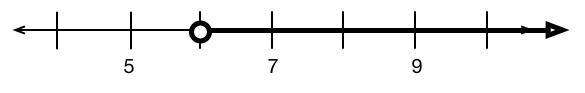# SAT Number Line

Algebra Level 2Which inequality is represented by the number line above ?

(A) $\ \ x > 5$
(B) $\ \ x > 6$
(C) $\ \ x > 7$
(D) $\ \ x \geq 6$
(E) $\ \ x < 6$

×

Problem Loading...

Note Loading...

Set Loading...